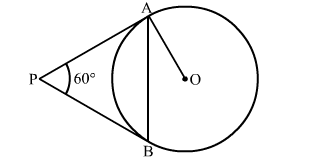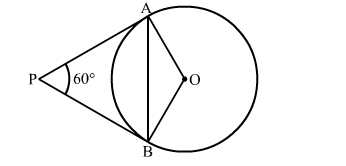# In the given figure, PA and PB are two tangents to a circle with centre O,`
Question:

In the given figure, PA and PB are two tangents to a circle  with centre O, If ∠APB = 60 then find the measure of ∠OABSolution:

Construction: Join OBWe know that the radius and tangent are perperpendular at their point of contact
∵∠OBP = ∠OAP = 90